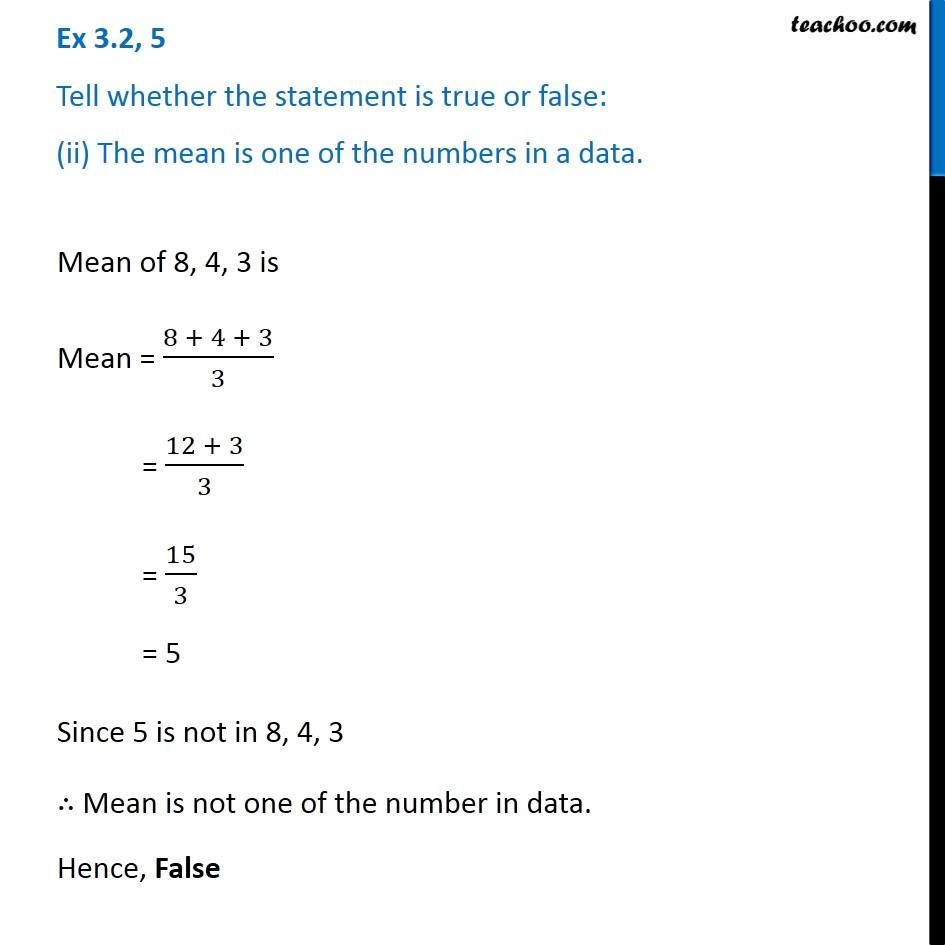Ex 3.2

Chapter 3 Class 7 Data Handling
Serial order wiseIntroducing your new favourite teacher - Teachoo Black, at only ₹83 per month

### Transcript

Ex 3.2, 5 Tell whether the statement is true or false: (ii) The mean is one of the numbers in a data. Mean of 8, 4, 3 is Mean = (8 + 4 + 3)/3 = (12 + 3)/3 = 15/3 = 5 Since 5 is not in 8, 4, 3 ∴ Mean is not one of the number in data. Hence, False